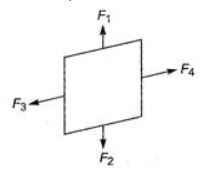# Please explain the following 31st question with reason.Dear Student
When a current carrying loop is placed in a magnetic field, the coil experiences a torque given by τ = NBi Asinθ. Torque is maximum when θ=90, i.e., the plane of the coil is parallel to the field τmax=NBiA.
Forces F→1 and F→2 acting on the coil are equal in magnitude and opposite in direction.
As the forces F→1 and F→2 have the same line of action their resultant effect on the coil is zero.
The two forces F→3 and F→4 are equal in magnitude and opposite in direction.
As the two forces have different lines of action, they constitute a torque.
Thus, if the force on one arc of the loop is F,  the net force on the remaining three arms of the loop is −F.Regards

• 0
What are you looking for?